19 Dates and Times
In the Wolfram Language, Now gives your current date and time.
 In:=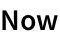Out=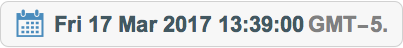Add a week to the current date and time:
 In:=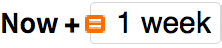Out=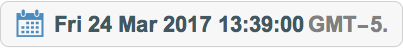Enter a date:
 In:=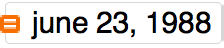Out=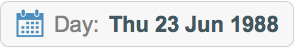Subtract two dates:
 In:=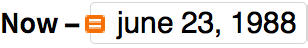Out=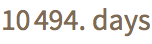In:=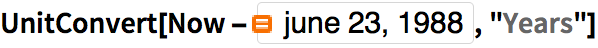Out=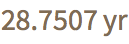DayRange is the analog of Range for dates:
 In:=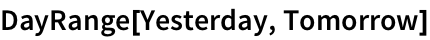Out=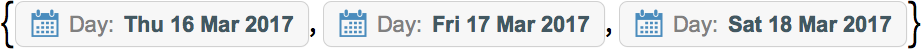DayName finds the day of the week for a particular date.
Compute the day of the week 45 days from now:
 In:=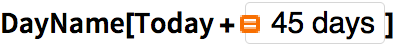Out=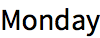Once you know a date, there are lots of things you can compute. For example, MoonPhase gives the phase of the moon (or, more accurately, the fraction of the Moon that is illuminated when seen from the Earth).
Compute the phase of the moon now:
 In:=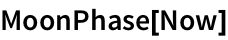Out=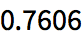Compute the phase of the moon on a certain date:
 In:=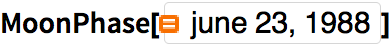Out=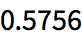Generate an icon for the phase of the moon:
 In:=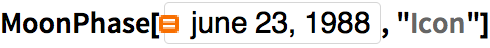Out=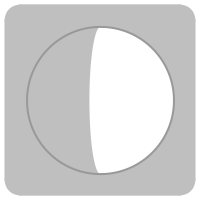If you know both the date and a location on Earth, you can work out when the sun will rise and set.
 In:=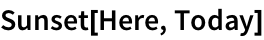Out=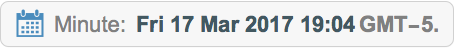In:=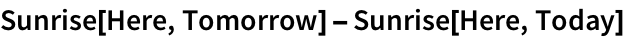Out=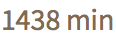Theyre about a minute off from being exactly 1 day (24 hours) apart:
 In:=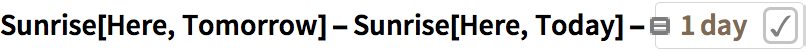Out=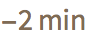Time zones are one of many subtleties. LocalTime gives the time in the time zone of a particular location.
 In:=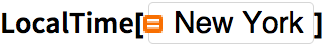Out=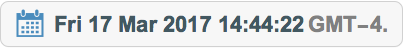In:=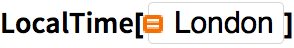Out=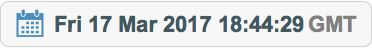Among the many areas where the Wolfram Language has extensive data is weather. The function AirTemperatureData uses this data to give the historical air temperature at a particular time and place.
Find the air temperature here at 6 pm yesterday:
 In:=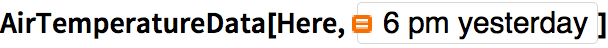Out=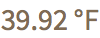If you provide a pair of dates, AirTemperatureData computes a time series of estimated temperatures between those dates.
Give a time series of air temperature measurements from a week ago until now:
 In:=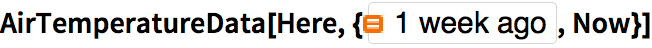Out=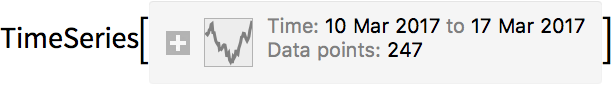DateListPlot is the analog of ListPlot for time series, where each value occurs at a particular date.
Plot the list of air temperature measurements:
 In:=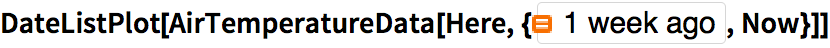Out=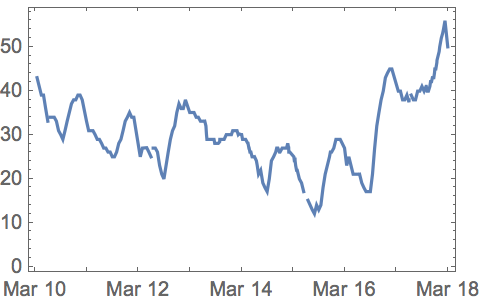As another example, lets look at data that goes much further back in time. WordFrequencyData tells one how frequently a particular word occurs, say in a sample of books published in a given year. Theres a lot of history one can see by looking at how this changes over the years and centuries.
 In:=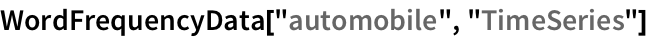Out=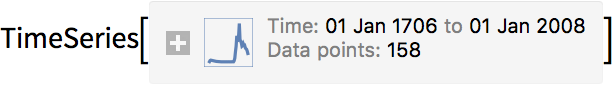Cars started to exist around 1900, but gradually stopped being called automobiles:
 In:=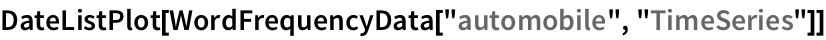Out=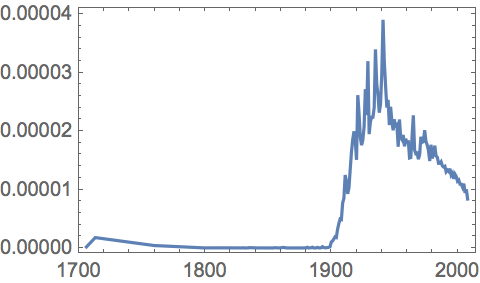WordFrequencyData is set up to make it easy to compare frequencies of different words. Lets see how monarchy and democracy have fared over the years. Democracy is definitely more popular now, but monarchy was more popular in the 1700s and 1800s.
Compare historical word frequency between monarchy and democracy:
 In:=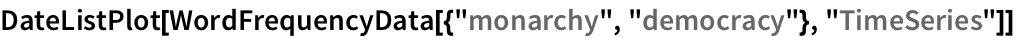Out=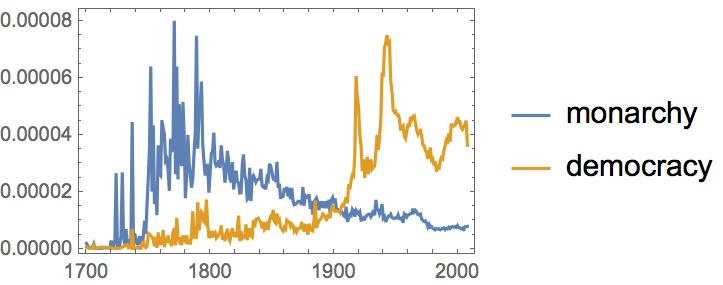19.1Compute how many days have elapsed since January 1, 1900. »
Sample expected output:
 Out[]=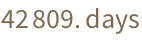Answer & check your solution
19.2Compute what day of the week January 1, 2000 was. »
Sample expected output:
 Out[]=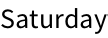Answer & check your solution
19.3Find the date a hundred thousand days ago. »
Sample expected output:
 Out[]=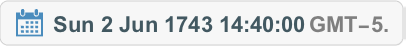Answer & check your solution
19.4Find the local time in Delhi. »
Sample expected output:
 Out[]=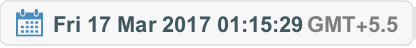Answer & check your solution
19.5Find the length of daylight today by subtracting todays sunrise from todays sunset. »
Sample expected output:
 Out[]=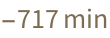Answer & check your solution
19.6Generate an icon for the current phase of the moon. »
Sample expected output:
 Out[]=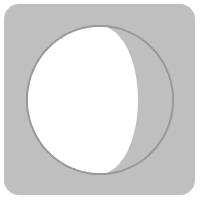Answer & check your solution
19.7Make a list of the numerical phase of the moon for each of the next 10 days. »
Sample expected output:
 Out[]=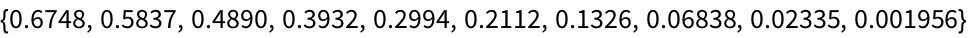Answer & check your solution
19.8Generate a list of icons for the moon phases from today until 10 days from now. »
Sample expected output:
 Out[]=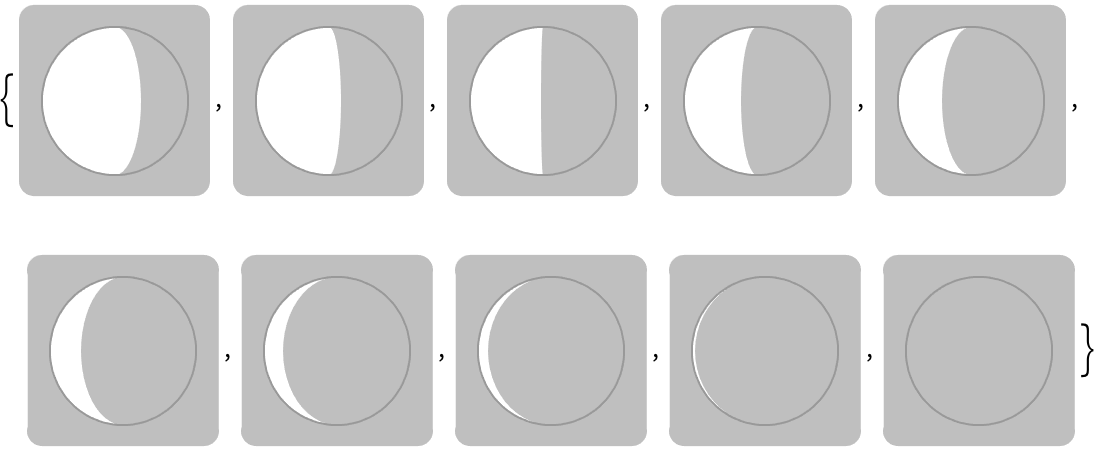Answer & check your solution
19.9Compute the time today between sunrise in New York City and in London. »
Sample expected output:
 Out[]=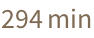Answer & check your solution
19.10Find the air temperature at the Eiffel Tower at noon yesterday. »
Sample expected output:
 Out[]=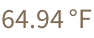Answer & check your solution
19.11Plot the temperature at the Eiffel Tower over the past week. »
Sample expected output:
 Out[]=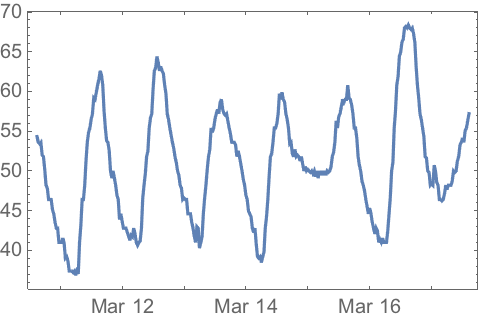Answer & check your solution
19.12Find the difference in air temperatures between Los Angeles and New York City now. »
Sample expected output:
 Out[]=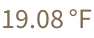Answer & check your solution
19.13Plot the historical frequency of the word groovy»
Expected output:
 Out[]=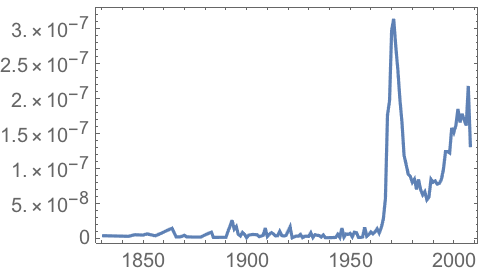Answer & check your solution
+19.1Compute how many weeks have elapsed since January 1, 1900. »
Sample expected output:
 Out[]=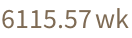Answer & check your solution
+19.2Compute the time between 3 pm today and sunset today. »
Sample expected output:
 Out[]=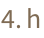Answer & check your solution
+19.3Generate an icon of the phase of the moon on August 29, 1959. »
Sample expected output:
 Out[]=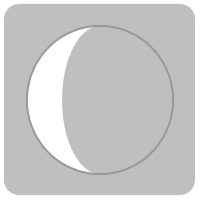Answer & check your solution
+19.4Make a line plot of the numerical phase of the moon for each of the next 30 days. »
Sample expected output:
 Out[]=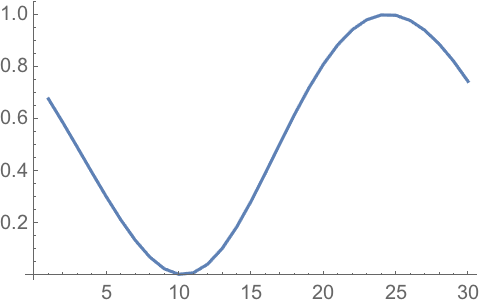Answer & check your solution
+19.5Make a Manipulate of the icon for the moon phase over the next 15 days. »
Sample expected output:
 Out[]=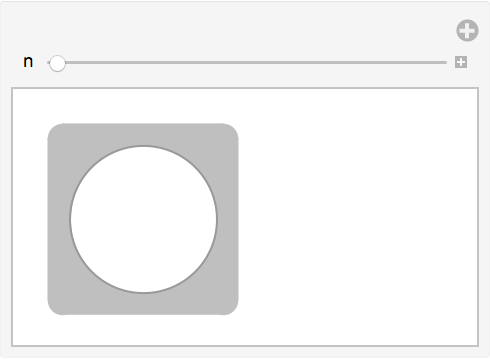Answer & check your solution
+19.6Show in a column the times of sunrise for 10 days, starting today. »
Sample expected output:
 Out[]=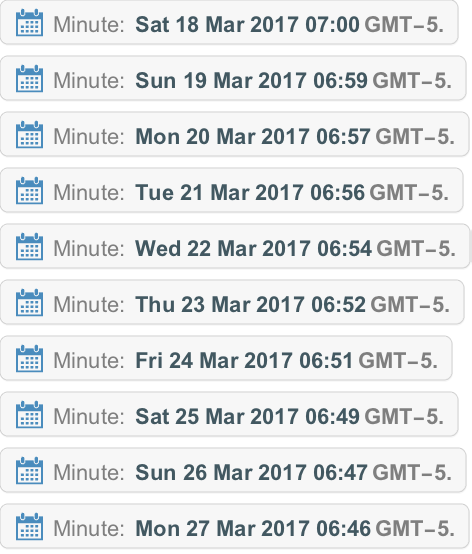Answer & check your solution
+19.7Plot together the historical frequencies of the words science and technology»
Expected output:
 Out[]=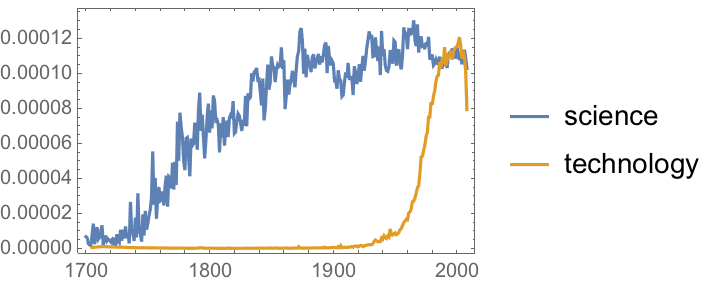Answer & check your solution
How can I get a date as a string?
Use DateString[date]. There are many options for the format of the string. For example, DateString[date, "DateShort"] uses short day and month names.
How can I extract the month or some other element from a date?
Use DateValue. DateValue[date, "Month"] gives the month number, DateValue[date, "MonthName"] gives the month name, etc.
How far in the past can dates be in the Wolfram Language?
Why are sunrise and sunset given only to the minute?
Because you cant compute more accurately than that exactly when the sun will actually rise and set without knowing things like air temperature that affect the bending of light in the Earths atmosphere.
Where does the Wolfram Language get air temperature data from?
What is a time series?
Its a way of specifying the values of something at a series of times. You can enter a time series in the Wolfram Language as TimeSeries[{{time1, value1}, {time2, value2}, ...}]. The Wolfram Language lets you do arithmetic and many other operations with time series.
What does DateListPlot do?
It plots values against times or dates. The values can be given in a TimeSeries[...] or in a list of the form {{time1, value1}, {time2, value2}, ...}.